## Monday, May 22, 2017

### `arc.cladelabels` using the names of descendant tips rather than the node numbers

Today a phytools blog reader commented:

“Liam, these clade labels are fantastic! But, I wonder if there is a possible way to determine clades by the tips (instead of by the nodes), since some clades could have just one branch.”

The answer is 'kind of.' That is, we can't give the function an argument that consists of the tip labels of our clade; however, we could specify the node using the descendant tips using a call to the function `findMRCA`.

For example:

``````library(phytools)
tree
``````
``````##
## Phylogenetic tree with 64 tips and 63 internal nodes.
##
## Tip labels:
##  t63, t64, t47, t48, t43, t44, ...
##
## Rooted; includes branch lengths.
``````
``````plotTree(tree,type="fan",fsize=0.8,xlim=c(-4.1,4.1))
``````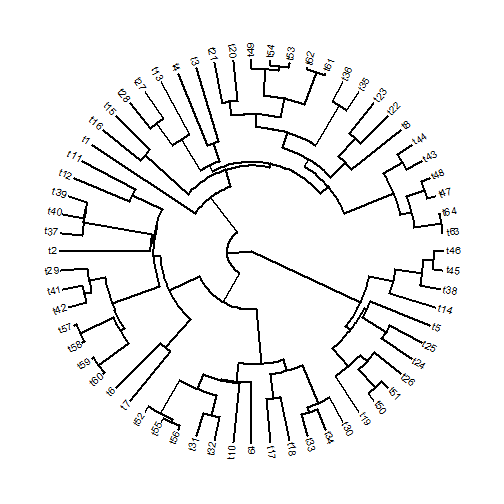If we want to label the clade containing t63, t64, t47, t48, t43, and t44 as clade A, then we can do it as follows:

``````plotTree(tree,type="fan",fsize=0.8,xlim=c(-4.1,4.1))
c("t63","t64","t47","t48","t43","t44")),ln.offset=1.1,
lab.offset=1.16)
``````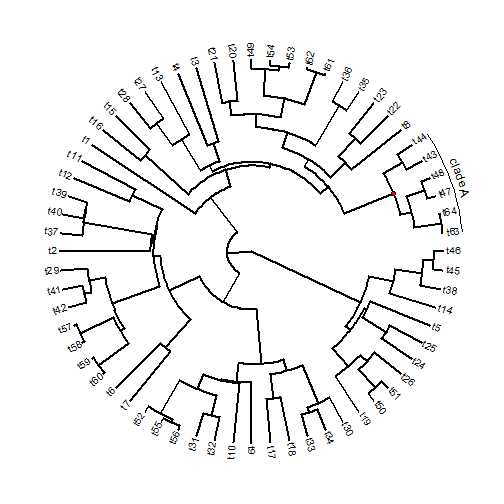We need not list all the taxa in the clade we want to label. For instance, if we want to label the clade containing taxa t46, t45, through t19, we only need list a set of tips for whom the MRCA is also the ancestor of the clade. For example:

``````plotTree(tree,type="fan",fsize=0.8,xlim=c(-4.1,4.1))
ln.offset=1.1,lab.offset=1.16)
## and our previous label:
c("t63","t64","t47","t48","t43","t44")),ln.offset=1.1,
lab.offset=1.16)
``````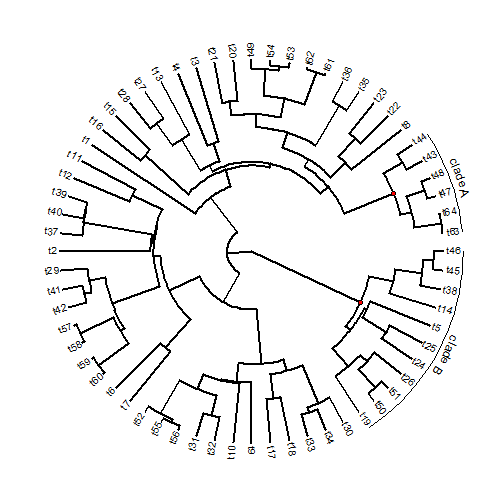Finally, if we want to label just one tip - well, we can just specify the node number of that tip. For instance to label the clade of tip t8 we can do:

``````plotTree(tree,type="fan",fsize=0.8,xlim=c(-4.1,4.1))
orientation="horizontal",ln.offset=1.1,lab.offset=1.12)
## with our other labels:
c("t63","t64","t47","t48","t43","t44")),ln.offset=1.1,
lab.offset=1.16)
ln.offset=1.1,lab.offset=1.16)
``````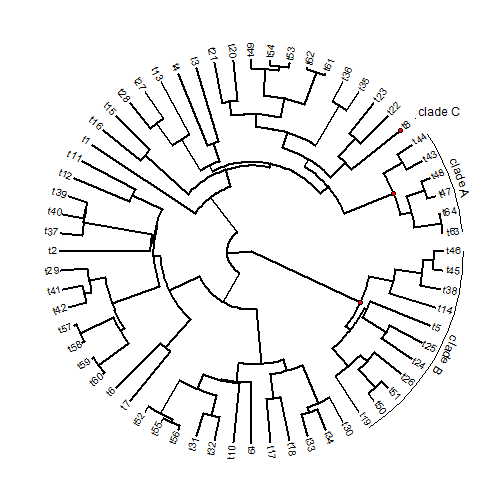Finally, those little red dots are just designed to keep track of the clade we are labeling. We can turn that off of course:

``````plotTree(tree,type="fan",fsize=0.8,xlim=c(-4.1,4.1),ftype="i")
c("t63","t64","t47","t48","t43","t44")),ln.offset=1.1,
lab.offset=1.16,mark.node=FALSE)
ln.offset=1.1,lab.offset=1.16,mark.node=FALSE)
orientation="horizontal",ln.offset=1.1,lab.offset=1.12,
mark.node=FALSE)
``````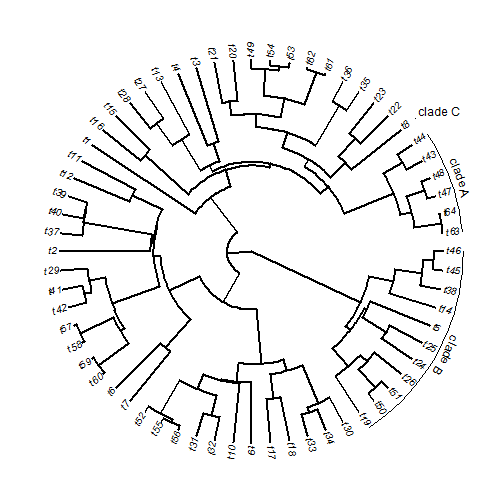That's it.

1.Hi Liam,

`arc.cladelabels` is a great function, have you test it's stability when the phylogenetic tree scaled up? Different errors occurred with the same code different OSs.
I have reported a issue [here](https://github.com/liamrevell/phytools/issues/79).

Thanks!
Miao

2.Hi,

How can I change the width of the line?

Note: due to the very large amount of spam, all comments are now automatically submitted for moderation.